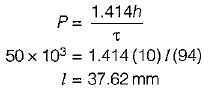Courses

# Test: Welded & Riveted Joints - 3

## 20 Questions MCQ Test Topicwise Question Bank for Mechanical Engineering | Test: Welded & Riveted Joints - 3

Description
This mock test of Test: Welded & Riveted Joints - 3 for Mechanical Engineering helps you for every Mechanical Engineering entrance exam. This contains 20 Multiple Choice Questions for Mechanical Engineering Test: Welded & Riveted Joints - 3 (mcq) to study with solutions a complete question bank. The solved questions answers in this Test: Welded & Riveted Joints - 3 quiz give you a good mix of easy questions and tough questions. Mechanical Engineering students definitely take this Test: Welded & Riveted Joints - 3 exercise for a better result in the exam. You can find other Test: Welded & Riveted Joints - 3 extra questions, long questions & short questions for Mechanical Engineering on EduRev as well by searching above.
QUESTION: 1

Solution:
QUESTION: 2

Solution:
QUESTION: 3

### According to IBR, the factor of safety of riveted joint should not be less than

Solution:
QUESTION: 4

Which one of the following is not an advantage of welded joints over riveted joint

Solution:
QUESTION: 5

The relationship between diameter of rivet hole and plate thickness (Unwin formula) is given by

Solution:

The relation between diameter of rivet hole and plate thickness is given by unwin as
d = 6√t
where, d = diameter of rivet hole plate thickness

QUESTION: 6

For longitudinal joint in boiler, the type of joint used is

Solution:

The longitudinal joint in a boiler shell is usually Butt joint with two cover plates this joint is more efficient than lap joint. It is also stiffer and helps to maintain circularity of the shell.

QUESTION: 7

The centre to centre distance between two consecutive rivets in a row is called

Solution: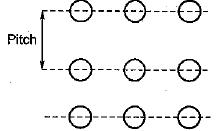QUESTION: 8

Strength of a rivet in bearing is given by
where
σb = bearing stress
σt = tensile stress
τ = shear stress

Solution:
QUESTION: 9

The objective of caulking in a riveted joint is to make the joint

Solution:

Caulking is used to make the riveted joint leak proof or fluid tight in pressure vessel like steam boilers, air receivers and tank etc.

QUESTION: 10

The important criterion in case of riveted joints for material storage and other ordinary tanks is

Solution:
QUESTION: 11

In a fillet welded joint, the weakest area of weld is

Solution:

Because throat area is the minimum area and Athroat = h x L

QUESTION: 12

A rivet in a structure shown in figure, has to support a load of 2 kN. If the primary and secondary shear stresses are 6.37 MPa and 13.50 MPa respectively, the maximum stress developed in the rivet is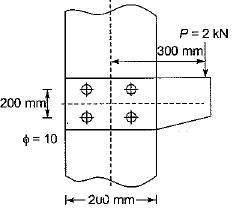Solution: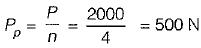Twisting moment = P x e
= 200 x 0.3 = 600 N-m
From the figure
r1 = r2 = r3 = r4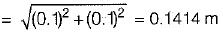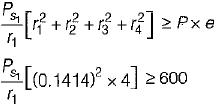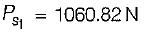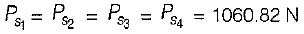Resultant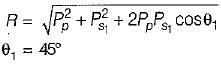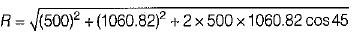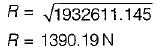shear stress developed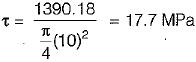QUESTION: 13

A riveted joint (with four rivets numbered 1 to 4) as shown in the figure is subjected to an eccentric loading. The least and the most stressed rivets respectively are: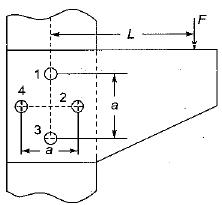Solution:

Since 2 is the nearest rivet to the line of action of the force hence, it will be most stressed rivet while 4 is very far from the line of action of the force hence it will be least stressed.

QUESTION: 14

If the ratio of diameter of rivet hole to the pitch of rivets is 0.25 then tearing efficiency of the joint is

Solution: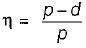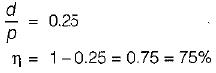QUESTION: 15

A bracket (shown in figure) is rigidly mounted on wall using four rivets. Each rivet is 6 mm in diameter and has an effective length of 12 mm. Direct shear stress (in MPa) in the most heavily loaded rivet is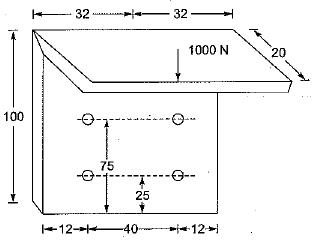Solution:

There are four rivets, direct force on each rivet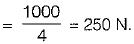Direct shear stress,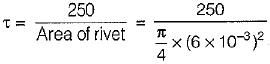= 8.8 MPa

QUESTION: 16

The diameter of a rivet will be if plate thickness is 8 mm

Solution:

d = 6√t = 6√8 = 16.9mm

QUESTION: 17

A double riveted double cover butt joint in plates 20 mm thick is made with 25 mm diameter rivets at 100 mm pitch. The permissible stresses are σ= 120 MPa, τ = 100 MPa, σc= 150 MPa. Taking the strength of the rivet in double shear as twice that of single shear, the tearing resistance of the plate is

Solution: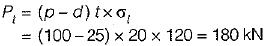QUESTION: 18

The permissible stress in a fillet weld is 100 N/mm2. The fillet weld has equal leg lengths of 15 mm each. The allowable shearing load on per cm length of the weld is

Solution:

Shear stress is given by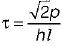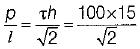∴ Load/cm length is = 1060 N/mm
= 10.6 kN/cm

QUESTION: 19

Two plates are joined together by means of single transverse and double parallel fillet welds as shown in the given figure. If the size of fillet is 5 mm and allowable shear load power mm is 300 N, what is the approximate length of each parallel fillet?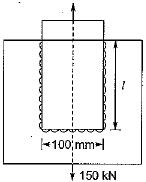Solution:

Total length of the weld = 2l+ 100
Allowable shear per mm is 300N
∴ 300 x (2l + 100) = 150 x 103
2l+ 100 = 500
l = 200 mm

QUESTION: 20

A steel plate 100 mm wide and 10 mm thick welded to another steel plate by means of double parallel fillet welds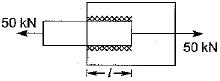Q. The plates are subjected to a static tensile force of 50 kN. If the permissible shear stress in the weld is 94 N/mm2. Then required length of the welds is

Solution: